# Math App Bundles

## Visual Fraction and Multiplication Table Bundle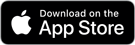Visual Fractions Decimals and Percentages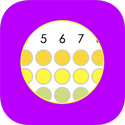Visual Multiplication TableFraction as Slope

## Math Division, Multiplication, Addition, Subtraction, Fractions, and RoundingFraction Math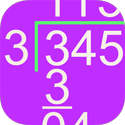Long DivisionPartial Quotients Division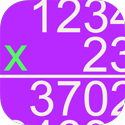Long Multiplication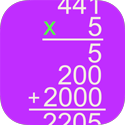Partial Products Multiplication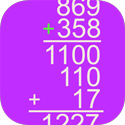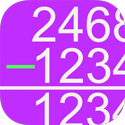Column Subtraction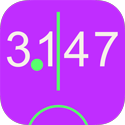Decimal RoundingWhole Number Rounding

## Order of Operations and Polynomial Algebra BundleOrder of Operations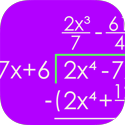Polynomial Long Division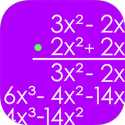Polynomial Multiplication## Alternative Multiplication and Addition Algorithms BundleVertically and Crosswise MultiplicationLattice Multiplication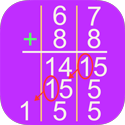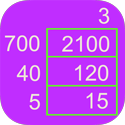Grid MultiplicationRussian MultiplicationEgyptian Multiplication

## Alternative Division and Subtraction Algorithms BundleSame-Change Subtraction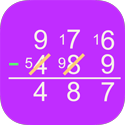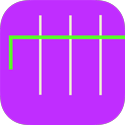Column Division

Counting-Up Subtraction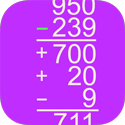Partial Differences Subtraction